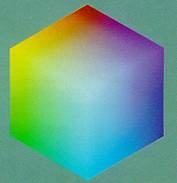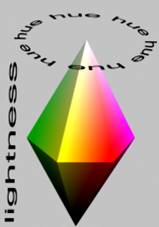CS420 Fall 2004 Homework, Week 6

Color spaces may be difficult to mentally ‘navigate’.  For instance it is difficult to have an intuitive feel for what color the RGB triple 256,80,45 identifies.

RGB color space:The HSL color space is more intuitive to most people since its dimensions correspond to parameters we typically use to describe color: hue, saturation, and luminance.

HSL Space:Hue is represented as an angle on the color circle with Red (arbitrarily) being 0° and the rest of the colors equally distributed around the circle (green=120°, blue=240°, etc.)

Saturation and luminance are represented as a percentage. 100% saturation is full saturation, 0% a shade of grey. Similarly 100% lightness is white and 0% lightness is black with 50% being ‘normal’.

For instance the primary color red would be (0°, 100%, 50%), (0°, 100%, 90%) and would be a pinkish tint.

1) Derive the formula for converting a HSL color to the corresponding RBG coordinate. (assume R, G, and B values are percentages, with 0% corresponding to no contribution of that color, and 100% being total contribution)

2) Derive the formula for converting a RGB color to the corresponding HSL coordinate. (same assumption of R, G, and B as percentages)

3) Create interactive program that allows you to navigate color space.  The program should numerically display a 24-bit (3 0-255 values) RGB triple, the corresponding HSL triple and a sample of the resulting color. Each parameter: R, G, B, H, S, and L should have a corresponding slider such that the position of the slider corresponds to the value of the parameter, and interactively moving the slider will simultaneously a) change the parameter, b) change the color displayed, c) update the sliders corresponding to the ‘other’ color space. Use the formulas derived in questions 1 and 2. Quantize the RGB values to display the color such that 0% corresponds to 0 and 100% corresponds to 100%.

Any interface toolkit may be used so long as the executable will run on my Windows XP SP2 machine without requiring other downloads.

Please submit the following for full credit:

-          Commented C/C++ code

-          Executable